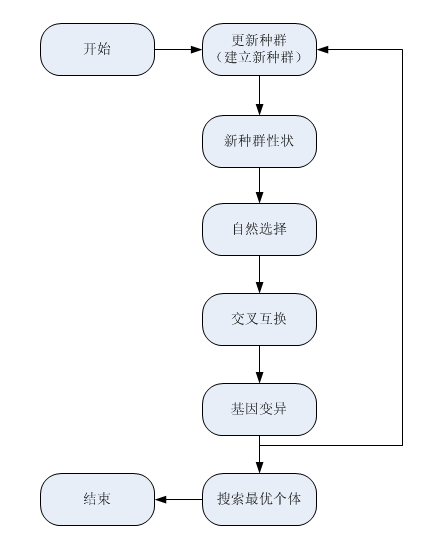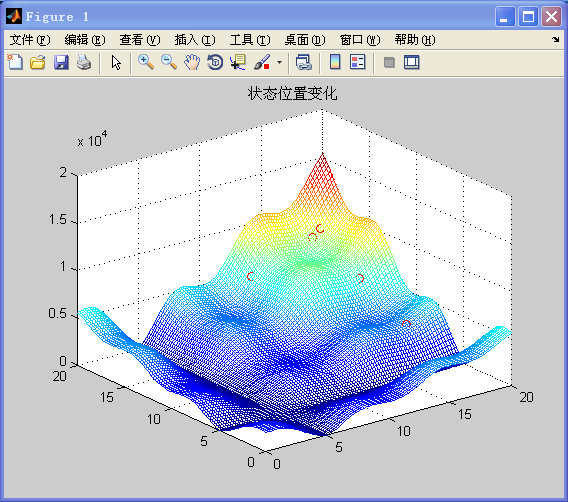遗传算法的原理和matlab实现（多维）clc;clear;close all;
%%
% f= @(a,b)(1/2*a .* sin(a) .* cos(1/2 * a) ).*(1/2*b .* sin(b) .* cos(1/2 * b) ); % 函数表达式
f= @(a,b)(10*sin(a)+7*abs(a-5)+10).*(10*sin(b)+7*abs(b-5)+10); % 函数表达式

figure(1);
[x0_1, x0_2]=meshgrid(0:.2:20);
y0=f(x0_1,x0_2);
mesh(x0_1, x0_2, y0);
hold on;

N = 50;                         % 初始种群个数
chromosome = 2;                  % 空间维数
ger = 50;                       % 最大迭代次数
chromlength =[16,16];
limit = [0, 20;0, 20];
pc = 0.3;                        % 交叉概率
pm = 0.008;                      % 变异概率

all_best=0;
all_x=0;

%%
% for i=1:chromosome
%     eval(['POP.chrom',num2str(i),'=round(rand(N,chromlength(i)));']);
%     for j=1:N
%         eval(['POP.x',num2str(i),'(j)=(binary2decimal(POP.chrom1(j,:)))/(2^chromlength(i)-1)*(limit(i,2)-limit(i,1)) ;'])
% %       POP.x'i'(j)=(binary2decimal(POP.chrom1(j,:)))/(2^chromlength(i)-1)*(limit(i,2)-limit(i,1)) ;
%     end
% end

POP.chrom1=round(rand(N,chromlength(1)));
POP.chrom2=round(rand(N,chromlength(2)));

%%
% 开始迭代
for n=1:ger
%%
% 交叉互换
for i = 1:2:N-1
if(rand<pc)
cpoint = round(rand*chromlength);
POP.NEWchrom1(i,:) = [POP.chrom1(i,1:cpoint(1)),POP.chrom1(i+1,cpoint(1)+1:chromlength(1))];
POP.NEWchrom1(i+1,:) = [POP.chrom1(i+1,1:cpoint(1)),POP.chrom1(i,cpoint(1)+1:chromlength(1))];
POP.NEWchrom2(i,:) = [POP.chrom2(i,1:cpoint(2)),POP.chrom2(i+1,cpoint(2)+1:chromlength(2))];
POP.NEWchrom2(i+1,:) = [POP.chrom2(i+1,1:cpoint(2)),POP.chrom2(i,cpoint(2)+1:chromlength(2))];
else
POP.NEWchrom1(i,:) = POP.chrom1(i,:);
POP.NEWchrom1(i+1,:) = POP.chrom1(i+1,:);
POP.NEWchrom2(i,:) = POP.chrom2(i,:);
POP.NEWchrom2(i+1,:) = POP.chrom2(i+1,:);
end
end
% 根据交叉互换的结果 更新种群的基因
POP.chrom1=POP.NEWchrom1;
POP.chrom2=POP.NEWchrom2;

%%
% 基因变异并更新种群
for i=1:N
for j1=1:chromlength(1)
if(rand<pm)
POP.chrom1(i,j1)=~POP.chrom1(i,j1);
end
end

for j2=1:chromlength(2)
if(rand<pm)
POP.chrom2(i,j2)=~POP.chrom2(i,j2);
end
end

end

%%
% 形状表达与选择

% 将基因(二进制编码)转化为自变量的取值（10进制的数）
for i=1:N
POP.x1(i)=(binary2decimal(POP.chrom1(i,:)))/(2^chromlength(1)-1)*(limit(1,2)-limit(1,1)) ;
end
for i=1:N
POP.x2(i)=(binary2decimal(POP.chrom2(i,:)))/(2^chromlength(2)-1)*(limit(2,2)-limit(2,1)) ;
end
% 根据自变量的取值得到函数的输出
for i=1:N
POP.y(i)=f(POP.x1(i),POP.x2(i));
end

%将输出的结果单位化，转化为0-1之间的数值长度（相当于轮盘赌的各个区域的面积）
a=min(POP.y);
b=sum(POP.y)+N*(-a);

for i=1:N
end

%数值长度转换为0-1之间的区间的节点（相当于把面积转化为了轮盘赌上各个区域的边界线）

for i=2:N
end

%进行轮盘赌，任取一个随机数cs，求这个随机数在轮盘赌中的位置区域
%到达某个区域，就代表下一个种群在第i个个体就拥有该区域所表示的基因，从而得到新种群
for i=1:N
cs=rand;
POP.NEWchrom1(i,:)=POP.chrom1(b,:);
POP.NEWchrom2(i,:)=POP.chrom2(b,:);
end

%更新种群
POP.chrom1=POP.NEWchrom1;
POP.chrom2=POP.NEWchrom2;

%%
% 获得最优位置
[this_x this_best]=best(POP)
if all_best<this_best
all_best=this_best;
all_x=this_x;
end
cla;
mesh(x0_1, x0_2, y0);
plot3(POP.x1,POP.x2,POP.y, 'ro');title('状态位置变化');
pause(0.1);
end

figure();
mesh(x0_1, x0_2, y0);
hold on;
plot3(all_x(1),all_x(2),all_best, 'ro');title('最优位置图');

disp(['最大值：',num2str(all_best)]);
disp(['变量取值：',num2str(all_x)]);


function [Nmin,Nmax]=POP_erfen(min,max,fun,tal)
if tal<fun(1)
Nmin=0;
Nmax=1;
else
a=min;
b=max;
if (b-a)<=1
Nmin=a;
Nmax=b;
else
c=round((a+b)/2);
if tal>=fun(c)
a=c;
b=b;
[Nmin,Nmax]=POP_erfen(a,b,fun,tal);
else
a=a;
b=c;
[Nmin,Nmax]=POP_erfen(a,b,fun,tal);
end
end
end
end


function [bestindividual bestfit] = best(POP)
[px,py] = size(POP.y);
bestindividual = [POP.x1(1) , POP.x2(1)];
bestfit = POP.y(1);
for i = 2:px
if POP.y(i)>bestfit
bestindividual = [POP.x1(i) , POP.x2(i)];
bestfit = POP.y(i);
end
end


%二进制转化成十进制函数
%输入变量：
%二进制种群
%输出变量
%十进制数值
function pop2 = binary2decimal(pop)
[px,py]=size(pop);
for i = 1:py
pop1(:,i) = 2.^(py-i).*pop(:,i);
end
%sum(.,2)对行求和，得到列向量
pop2 = sum(pop1,2);（后面打算把整个过程用GIF图表现出来，未完待续）